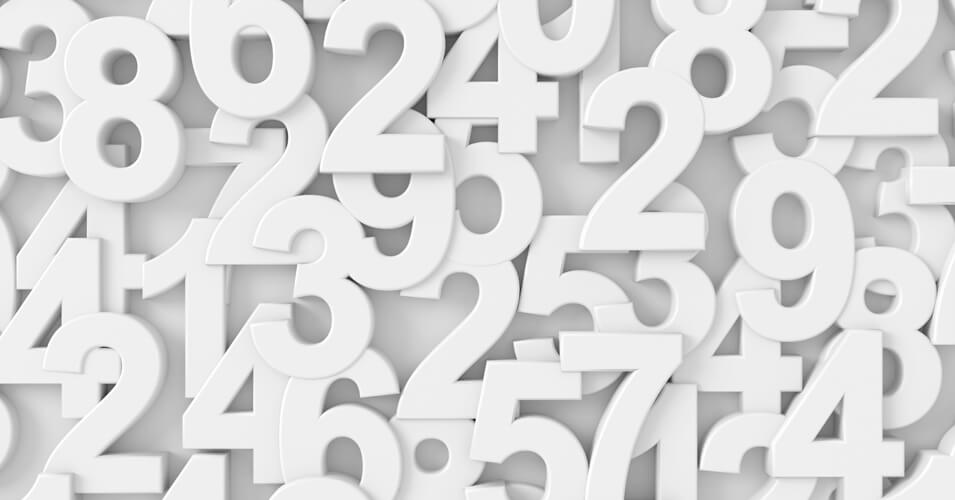• Save

# Numerical Ability Questions and Answers for Bank ExamThe level of Numerical Aptitude in Banking exams is becoming very difficult, so the student is taking a lot of time to solve these questions. So, here I am answering important numerical ability questions for you, through which practice you can check your level.

With practice these numerical ability questions and answers are given here, you can obtain the best marks in the examination. According to these questions answers, you can improve your mental ability and can get the score high in your exam.

For more practice of numerical aptitude test questions, you can visit here.

## Numerical Ability Questions with Answers:

Q.1. In covering a distance of 30 km, Abhay takes 2 hours more than Sameer. If Abhay doubles his speed, then he would take 1 hour less than Sameer. Abhay's speed is:

(A) 5 kmph

(B) 6 kmph

(C) 6.25 kmph

(D) 7.5 kmph

Ans .   A

Q.2. Tea worth of Rs. 135/kg & Rs. 126/kg are mixed with a third variety in the ratio 1: 1 : 2. If the mixture is worth Rs. 153 per kg, the price of the third variety per kg will be____?

(A) Rs. 169.50

(B) Rs.1700

(C) Rs. 175.50

(D) Rs. 180

Ans .   C

Q.3. Robert is travelling on his cycle and has calculated to reach point A at 2 P.M. if he travels at 10 kmph, he will reach there at 12 noon if he travels at 15 kmph. At what speed must he travel to reach A at 1 P.M.?

(A) 8 kmph

(B) 11 kmph

(C) 12 kmph

(D) 14 kmph

Ans .   C

Q.4. 8 litres are drawn from a cask full of wine and is then filled with water. This operation is performed three more times. The ratio of the quantity of wine now left in cask to that of the water is 16 : 65. How much wine the cask hold originally?

(A) 18 litres

(B) 24 litres

(C) 32 litres

(D) 42 litres

Ans .   B

Q.5. How many seconds will a 500-metre-long train take to cross a man walking with a speed of 3 km/hr in the direction of the moving train if the speed of the train is 63 km/hr?

(A) 25

(B) 30

(C) 40

(D) 45

Ans .   B

Q.6. A can contains a mixture of two liquids A and B in the ratio 7 : 5. When 9 litres of mixture are drawn off and the can is filled with B, the ratio of A and B becomes 7 : 9. How many litres of liquid A was contained by the can initially?

(A) 10

(B) 20

(C) 21

(D) 25

Ans .   C

Q.7. The average of 20 numbers is zero. Of them, at the most, how many may be greater than zero?

(A) 0

(B) -1

(C) 1

(D) none of these

Ans .   A

Q.8. Two goods train each 500 m long, are running in opposite directions on parallel tracks. Their speeds are 45 km/hr and 30 km/hr respectively. Find the time taken by the slower train to pass the driver of the faster one.

(A) 12 sec

(B) 24 sec

(C) 48 sec

(D) 60 sec

Ans .   B

Q.9. If log 64 = 1.8061, then the value of log 16 will be ( approx )?

(A) 1.9048

(B) 1.2040

(C) 0.9840

(D) 1.4521

Ans .   B

Q.10. A, B and C can do a piece of work in 20, 30 and 60 days respectively. In how many days can A do the work if he is assisted by B and C on every third day?

(A) 12 days

(B) 15 days

(C) 16 days

(D) 18 days

Ans .   B

If you have any doubt regarding numerical ability questions and answers, you can ask me in the comment section. Visit on the next page for more practice of these questions